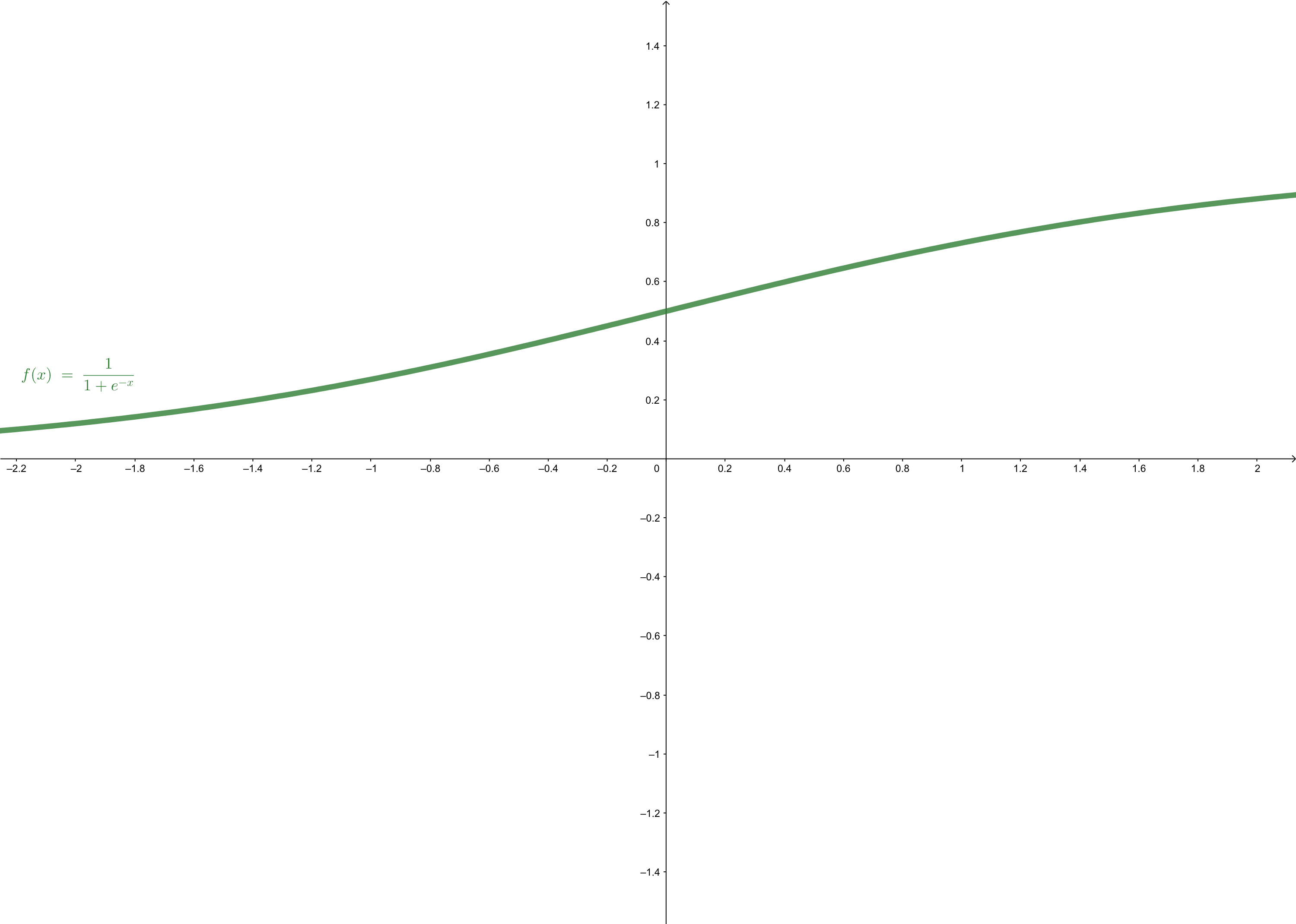## Preliminaries#

1. Probability
• Bayesian Formular
1. Calculus

## Probabilistic Generative Models1#

The generative model used for making decisions contains an inference step and a decision step:

1. Inference step is to calculate $$\Pr(\mathcal{C}_k|\mathbf{x})$$ which means the probability of $$\mathbf{x}$$ belonging to the class $$\mathcal{C}_k$$ given $$\mathbf{x}$$
2. Decision step is to make a decision based on $$\Pr(\mathcal{C}_k|\mathbf{x})$$ which was calculated in step 1

In this post, we just give an introduction and a framework for the probabilistic generative model in classification. But the details of how to estimate the parameters in the model will not be introduced.

## From Bayesian Formular to Logistic Sigmoid Function#

To build $$\Pr(\mathcal{C}_k|\mathbf{x})$$, we can start from Bayesian formula. To the class $$\mathcal{C}_1$$ of a two-classes problem, the posterior probability:

\begin{aligned} \Pr(\mathcal{C}_1|\mathbf{x})&=\frac{\Pr(\mathbf{x}|\mathcal{C}_1)\Pr(\mathcal{C}_1)}{\Pr(\mathbf{x}|\mathcal{C}_1)\Pr(\mathcal{C}_1)+\Pr(\mathbf{x}|\mathcal{C}_2)\Pr(\mathcal{C}_2)}\\ &=\frac{1}{1+\frac{\Pr(\mathbf{x}|\mathcal{C}_2)\Pr(\mathcal{C}_2)}{\Pr(\mathbf{x}|\mathcal{C}_1)\Pr(\mathcal{C}_1)}} \end{aligned}\tag{1}

represents a new function:

\begin{aligned} \Pr(\mathcal{C}_1|\mathbf{x})&=\delta(a)\\ &=\frac{1}{1+e^{-a}} \end{aligned}\tag{2}

where:

$a=\ln\frac{\Pr(\mathbf{x}|\mathcal{C}_1)\Pr(\mathcal{C}_1)}{\Pr(\mathbf{x}|\mathcal{C}_2)\Pr(\mathcal{C}_2)}\tag{3}$

An usual question is why we set $$a=\ln\frac{\Pr(\mathbf{x}|\mathcal{C}_1)\Pr(\mathcal{C}_1)}{\Pr(\mathbf{x}|\mathcal{C}_2)\Pr(\mathcal{C}_2)}$$ but not $$a=\ln\frac{\Pr(\mathbf{x}|\mathcal{C}_2)\Pr(\mathcal{C}_2)}{\Pr(\mathbf{x}|\mathcal{C}_1)\Pr(\mathcal{C}_1)}$$. In my opinion, this $$a$$ just determine the graph of function $$\delta(a)$$. However, we perfer monotone-increasing function and $$\frac{1}{1+e^{-a}}$$ is just a monotone-increasing function but $$\frac{1}{1+e^{a}}$$ is not.

$$\delta(\cdot)$$ is called logistic sigmoid function or squashing function, because it maps any number into interval $$(0,1)$$. The range of the function is just within the range of probability. So it is a good way to represent some kinds of probability, such as the $$\Pr(\mathcal{C}_1|\mathbf{x})$$. the shape of the logistic sigmoid function is:## Some Properties of Logistic Sigmoid#

For the logistic sigmoid function is symmetrical, then:

$1-\delta(a)=\frac{e^{-a}}{1+e^{-a}}=\frac{1}{e^a+1}\tag{4}$

and:

$\delta(-a)=\frac{1}{1+e^a}\tag{5}$

So, we have an important equation:

$1-\delta(a)=\delta(-a)\tag{6}$

The inverse function of $$y=\delta(a)$$ is: \begin{aligned} y&=\frac{1}{1+e^{-a}}\\ e^{-a}&=\frac{1}{y}-1\\ a&=-\ln(\frac{1-y}{y})\\ a&=\ln(\frac{y}{1-y}) \end{aligned}\tag{7}

The derivative of the logistic sigmoid function is: $\frac{d\delta(a)}{d a}=\frac{e^{-a}}{(1+e^{-a})^2}=(1-\delta(a))\delta(a)\tag{8}$

## Multiple Classes Problems#

We, now, extend the logistic sigmoid function into multiple classes condition. And we also start from the Bayesian formula:

$\Pr(\mathcal{C}_k|\mathbf{x})=\frac{\Pr(\mathbf{x}|\mathcal{C}_k)\Pr(\mathcal{C}_k)}{\sum_i\Pr(\mathbf{x}|\mathcal{C}_i)\Pr(\mathcal{C}_i)}\tag{9}$

In this condition,if we set $$a_i=\ln\frac{\Pr(\mathbf{x}|\mathcal{C}_k)\Pr(\mathcal{C}_k)}{\Pr(\mathbf{x}|\mathcal{C}_i)\Pr(\mathcal{C}_i)}$$, the whole fomular will be too complecated. To simplify the equation, we just set:

$a_i=\ln \Pr(\mathbf{x}|\mathcal{C}_k)\Pr(\mathcal{C}_k)\tag{10}$

and we get a function of posterior probability: $\Pr(\mathcal{C}_k|\mathbf{x})=\frac{e^{a_k}}{\sum_i e^{a_i}}\tag{11}$ And according to the property of probability, we get the value of function: $y(a)=\frac{e^{a_k}}{\sum_i e^{a_i}}\tag{12}$ belongs to interval $$[0,1]$$. And it is called the softmax function. Although according to equation (10), the domain of the definition of softmax function is $$(-\infty,0]$$, $$a$$ can be any real number. It’s called softmax because it is a smooth version of the max function.

When $$a_k\gg a_j$$ for $$k\neq j$$, we have:

\begin{aligned} \Pr(\mathbf{x}|\mathcal{C}_k)&\simeq1\\ \Pr(\mathbf{x}|\mathcal{C}_j)&\simeq0 \end{aligned}

So both the logistic sigmoid function and softmax function can be used to form generative classifiers, which gives a value to the decision step.

1. Bishop, Christopher M. Pattern recognition and machine learning. springer, 2006.↩︎